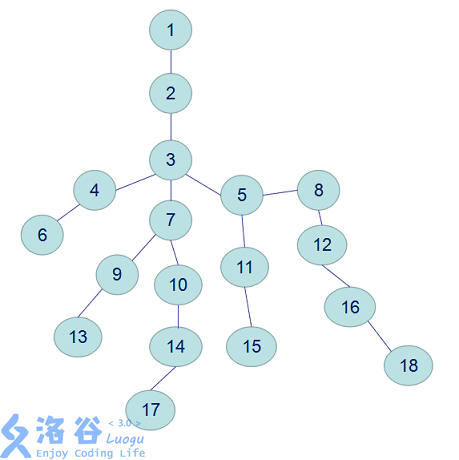# MorsLin 的博客# MorsLin 的博客

### 题解 P3379 【【模板】最近公共祖先（LCA）】

posted on 2018-03-21 19:30:22 | under 题解 |

LCA（Least Common Ancestors），即最近公共祖先，是指在有根树中，找出某两个结点u和v最近的公共祖先。 ———来自百度百科• 暴力算法

以 $17$ 和 $18$ 为例，既然要求LCA，那么我们就让他们一个一个向上爬(我要一步一步往上爬 —— 《蜗牛》)，直到相遇为止。第一次相遇即是他们的LCA。 模拟一下就是：
$17->14->10->7->3$
$18->16->12->8->5->3$
最终结果就是 $3$
当然这个算法妥妥的会T飞掉，那么我们就要进行优化，于是就有了用倍增来加速的倍增LCA，这也是我们今天介绍的重点。

• 倍增算法

所谓倍增，就是按$2$的倍数来增大，也就是跳 $1,2,4,8,16,32$ …… 不过在这我们不是按从小到大跳，而是从大向小跳，即按……$32,16,8,4,2,1$来跳，如果大的跳不过去，再把它调小。这是因为从小开始跳，可能会出现“悔棋”的现象。拿 $5$ 为例，从小向大跳，$5≠1+2+4$,所以我们还要回溯一步，然后才能得出$5=1+4$；而从大向小跳，直接可以得出$5=4+$1。这也可以拿二进制为例，$5(101)$，从高位向低位填很简单，如果填了这位之后比原数大了，那我就不填，这个过程是很好操作的。

还是以 $17$ 和 $18$ 为例，如果分别从$17$和$18$跳到$3$的话，它们的路径分别是（此例只演示倍增，并不是倍增LCA算法的真正路径）
$17->3$
$18->5->3$

可以看出向上跳的次数大大减小。这个算法的时间复杂度为$O(nlogn)$,已经可以满足大部分的需求。

想要实现这个算法，首先我们要记录各个点的深度和他们$2^i$级的的祖先，用数组$\rm{depth}$表示每个节点的深度，$fa[i][j]$表示节点$i$的$2^j$级祖先。 代码如下：

void dfs(int now, int fath) {  //now表示当前节点，fath表示它的父亲节点
fa[now] = fath; depth[now] = depth[fath] + 1;
for(int i = 1; i <= lg[depth[now]]; ++i)
fa[now][i] = fa[fa[now][i-1]][i-1]; //这个转移可以说是算法的核心之一
//意思是now的2^i祖先等于now的2^(i-1)祖先的2^(i-1)祖先
//2^i = 2^(i-1) + 2^(i-1)
for(int i = head[now]; i; i = e[i].nex)
if(e[i].t != fath) dfs(e[i].t, now);
}

预处理完毕后，我们就可以去找它的LCA了，为了让它跑得快一些，我们可以加一个常数优化(来自洛谷提高组讲义)

for(int i = 1; i <= n; ++i) //预先算出log_2(i)+1的值，用的时候直接调用就可以了
lg[i] = lg[i-1] + (1 << lg[i-1] == i);  //看不懂的可以手推一下

接下来就是倍增LCA了，我们先把两个点提到同一高度，再统一开始跳。

但我们在跳的时候不能直接跳到它们的LCA，因为这可能会误判，比如$4$和$8$，在跳的时候，我们可能会认为$1$是它们的LCA，但$1$只是它们的祖先，它们的LCA其实是$3$。所以我们要跳到它们LCA的下面一层，比如$4$和$8$，我们就跳到$4$和$5$，然后输出它们的父节点，这样就不会误判了。

int LCA(int x, int y) {
if(depth[x] < depth[y]) //用数学语言来说就是：不妨设x的深度 >= y的深度
swap(x, y);
while(depth[x] > depth[y])
x = fa[x][lg[depth[x]-depth[y]] - 1]; //先跳到同一深度
if(x == y)  //如果x是y的祖先，那他们的LCA肯定就是x了
return x;
for(int k = lg[depth[x]] - 1; k >= 0; --k) //不断向上跳（lg就是之前说的常数优化）
if(fa[x][k] != fa[y][k])  //因为我们要跳到它们LCA的下面一层，所以它们肯定不相等，如果不相等就跳过去。
x = fa[x][k], y = fa[y][k];
return fa[x];  //返回父节点
}

完整的求$17$和$18$的LCA的路径：
$17->10->7->3$
$18->16->8->5->3$
解释：首先，$18$要跳到和$17$深度相同，然后$18$和$17$一起向上跳，一直跳到LCA的下一层($17$是$7$，$18$是$5$)，此时LCA就是它们的父亲

#### 完整代码：

#include <iostream>
#include <cstdio>
#include <cstring>
#include <algorithm>
using namespace std;
struct zzz {
int t, nex;
}e[500010 << 1]; int head, tot;
void add(int x, int y) {
e[++tot].t = y;
}
int depth, fa, lg;
void dfs(int now, int fath) {
fa[now] = fath; depth[now] = depth[fath] + 1;
for(int i = 1; i <= lg[depth[now]]; ++i)
fa[now][i] = fa[fa[now][i-1]][i-1];
for(int i = head[now]; i; i = e[i].nex)
if(e[i].t != fath) dfs(e[i].t, now);
}
int LCA(int x, int y) {
if(depth[x] < depth[y]) swap(x, y);
while(depth[x] > depth[y])
x = fa[x][lg[depth[x]-depth[y]] - 1];
if(x == y) return x;
for(int k = lg[depth[x]] - 1; k >= 0; --k)
if(fa[x][k] != fa[y][k])
x = fa[x][k], y = fa[y][k];
return fa[x];
}
int main() {
int n, m, s; scanf("%d%d%d", &n, &m, &s);
for(int i = 1; i <= n-1; ++i) {
int x, y; scanf("%d%d", &x, &y);
}